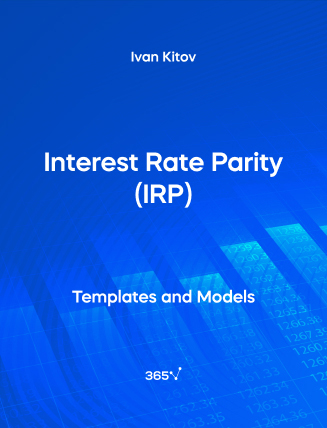## Interest Rate Parity (IRP) – Excel Template###### Ivan Kitov

The interest rate parity (IRP) suggests that the interest rate differential between two countries equals the differential between the forward exchange rate and the spot exchange rate. In this way, the concept assumes a strong relationship between spot rates, interest rates, and forward rates. You can use the interest rate parity to calculate the forward exchange rate—simply multiply the spot currency rate by the domestic currency interest rate, and divide it by the foreign currency interest rate.

This open-access Excel template is a useful tool for bankers, investment professionals, corporate finance practitioners, and portfolio managers.

Interest Rate Parity (IRP) is among the topics included in the Economics module of the CFA Level 1 Curriculum. Gain valuable insights into the subject with our Macroeconomics course.

You can also explore other related templates such as—Net Present Value (NPV), and Present Value (PV).Top